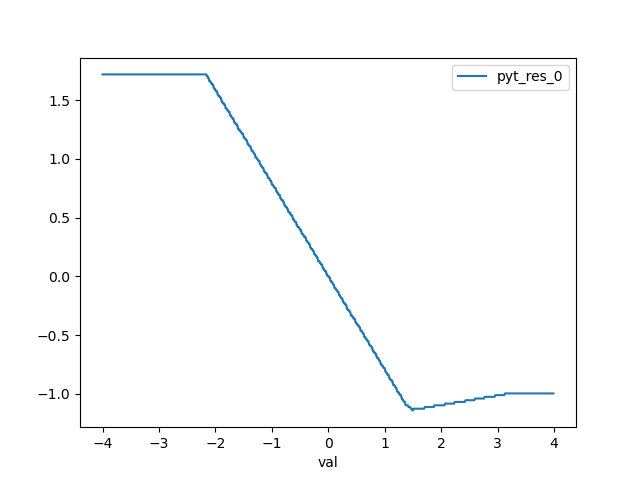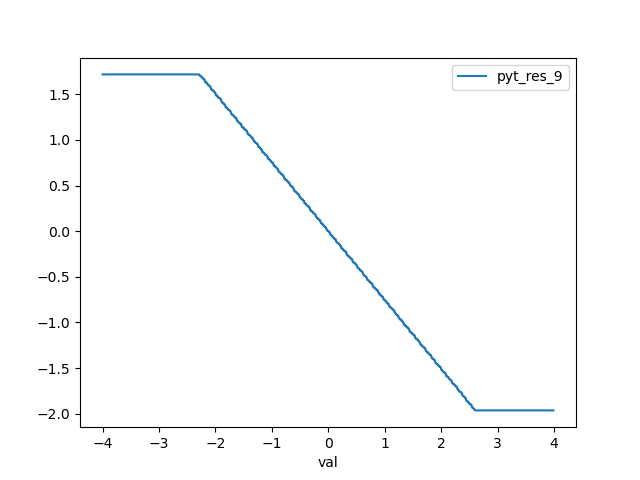# Is there anything wrong with the Quantization Math?

Hi,

I have been working on quantization for a while and while working on a project in which I was planning to perform the more efficient bit-shift rescaling instead of a float multiplication, I have noticed some discrepancies with my mathematical model. After digging deeper in the implementation of my code, PyTorch and FBGEMM, I made some tests to check where the source of the error lies.

For the test I ran the following code:

``````import torch
import random
import numpy as np
import pandas as pd

from collections import defaultdict
from matplotlib import pyplot as plt
from torch.quantization.observer import MinMaxObserver

SEED = 100
np.random.seed(SEED)
torch.manual_seed(SEED)
random.seed(SEED)

SHAPE_IN = 10
SHAPE_OUT = 10
BS = 100

MIN = -4
MAX = 4

class M(torch.nn.Module):
def __init__(self):
super(M, self).__init__()
# QuantStub converts tensors from floating point to quantized
self.quant = torch.quantization.QuantStub()
self.fc = torch.nn.Linear(SHAPE_IN, SHAPE_OUT, bias=False)
# DeQuantStub converts tensors from quantized to floating point
self.dequant = torch.quantization.DeQuantStub()

def forward(self, inp):
# manually specify where tensors will be converted from floating
# point to quantized in the quantized model
inp = self.quant(inp)
x = self.fc(inp)
# manually specify where tensors will be converted from quantized
# to floating point in the quantized model
x = self.dequant(x)
return x

def main():
# create a model instance
model_fp32 = M()

# model must be set to eval mode for static quantization logic to work
model_fp32.eval()

# attach a global qconfig, which contains information about what kind
# of observers to attach. Use 'fbgemm' for server inference and
# 'qnnpack' for mobile inference. Other quantization configurations such
# as selecting symmetric or assymetric quantization and MinMax or L2Norm
# calibration techniques can be specified here.
model_fp32.qconfig = torch.quantization.QConfig(
activation=MinMaxObserver.with_args(dtype=torch.quint8),
weight=MinMaxObserver.with_args(dtype=torch.qint8)
)

# Prepare the model for static quantization. This inserts observers in
# the model that will observe activation tensors during calibration.
model_fp32_prepared = torch.quantization.prepare(model_fp32)

# calibrate the prepared model to determine quantization parameters for activations
# in a real world setting, the calibration would be done with a representative dataset
input_fp32 = torch.randn((BS, SHAPE_IN))
model_fp32_prepared(input_fp32)

# Convert the observed model to a quantized model. This does several things:
# quantizes the weights, computes and stores the scale and bias value to be
# used with each activation tensor, and replaces key operators with quantized
# implementations.
model_int8 = torch.quantization.convert(model_fp32_prepared)

return model_int8

def test(val, model_int8):

input_fp32 = torch.ones(
(BS, SHAPE_IN)) * val # constant tensor with entries = val

# compute quantized result with pytorch quantization
pytorch_res = model_int8(input_fp32)

return pytorch_res

def make_plot():
d = defaultdict(list)
model_int8 = main()
for val in np.arange(MIN, MAX, 0.01):
pyt_res = test(val, model_int8)
d['val'].append(val)
for i in range(SHAPE_OUT):
d[f'pyt_res_{i}'].append(pyt_res[i].item())

figsize = (1, 25)
for i in range(SHAPE_OUT):
plt.figure(figsize=figsize)
df_a = pd.DataFrame(data={k: v for k, v in d.items() if k in ['val', f'pyt_res_{i}']})
df_a.plot(x='val')
plt.savefig(f"./experimental/res_{i}.png")
plt.close()

if __name__ == '__main__':
make_plot()
``````

The operation performed here is basically a single matrix-vector product. Which was even simplified to have the inputs with all the same values. This means that the operation can be seen as:

``````Y = WX = W [val ,val, val]^T = torch.sum(W, dim= 1) * val
``````

Example:

``````W = [[ 1, 10],
[15,  7]|

X = [5, 5]^T

Y = WX = [11, 22]^T * 5 = [55, 110]^T
``````

In my example what I am doing is the following, given a NN, I prepare it for quantization using 100 example datapoints. After converting it, I generate datapoints in the range [-4, 4] and probe the NN and check the output of ever feature.

EXPECTED BEHAVIOUR:
Given the math, and the nature of the inputs (single value). The output of the network for each feature should be monotonic. This means it should look like a clamped ramp (either negative or positive depending on the weight).

RESULTS and OBSERVATIONS:
To have an additional control, I checked also how TFLite behaves. My model and TF show for the same test the same behaviour. The output starts from the boundary of the range, when increasing the value of the input the output starts increasing/decreasing linearly until it reaches the other boundary of the range. So far so good.

PyTorch instead does not she the same behaviour. At least, it does not show that for `SHAPE_IN > 1`. Here are some plots:• pyt_res_9 is the 10th output feature and shows the correct trend (the slope could be positive as well)
• pyt_res_0 is the 1st output feature and shows a change of slope at a certain point in the range
• pyt_res_3 is the 4th output feature and shows twice a change of slope in the range

What is going on? Is this a bug or is there some intended behaviour I am not aware about?

3 Likes

Sorry for the late reply, first of all, we do have tests for correctness of our quantized linear implementations: pytorch/test_quantized_op.py at master · pytorch/pytorch · GitHub

For the problems you are seeing above, I’m not exactly sure if we would expect a monotonic increasing or decreasing trend. I think quantization itself is not a linear operation since it has clamping, also in calibration time you are calibration with torch.randn(((BS, SHAPE_IN)) and in test time you are testing with torch.ones(…) * val maybe that could be problematic as well.

1 Like

if you do:

torch.backends.quantized.engine=‘qnnpack’

this phenomenon goes away

if you do:

torch.backends.quantized.engine=‘fbgemm’ (which is the default qengine)

and in the observer (the activation one) use reduce_range=True

then the phenomenon should go away there too

basically the fbgemm quantized linear kernels have an overflow issue which causes the weird behavior you are seeing. This is why the default qconfig for fbgemm has reduce_range=True

1 Like

Dear @jerryzh168 and @HDCharles. Thank you very much for your replies. The problem was indeed in FBGEMM. When changing the backend to QNNPACK I could get the results to match across the different references.

@jerryzh168 I am sorry I didn’t reply earlier to your post. I was writing a detailed reply that was taking longer than expected, but in the meantime @HDCharles answered, and then checked right away if that was the solution to my problem.

@HDCharles thank you very much for the solution. Although, I cannot really understand where the error comes from. I check the C++ implementation of the Linear layer, quantization and so on, and couldn’t find the source of the overflow. Also, I could see that behaviour also when the input shape was 2, meaning that 2 multiplications and an accumulation were leading to overflow. But I cannot see how this can be possible given that the operations are performed in int32, and that specific operations required 17 bits to store the result in full precision (assuming zero_point = 0). I.e., `8bits multiplication -> 16 bits, 16bits accumulation of 2 elements -> 17 bits`

Also, if due to an overflow, I would expect the plots to be discontinuous (127 + 1 = -128), on the other hand, the plots are continuous and there are changes in the slope.

Where does the overflow originate from? Is it an intended behaviour?

You’d have to ask the fbgemm developers, our team doesn’t do much with the kennels, we focus more on the model transformation piece.

If I were to guess though, I think it doesnt wrap when it overflows, it stays at that value. So if you have ab+ac, as a increases one of those terms reaches a maximum and then stays constant but the other keeps changing. This can fully explain the plots given the following 3 cases:

1. If ba and ca are both saturated, the value stays constant

2. if one is saturated but b and c have the same sign, the slope gets shallower after the saturation point

3. if one is saturated but b and c have different signs then the first saturation point will be a local min/max.

Although it accumulates to int32 I’m guessing the initial int8*int8 single element multiplies only accumulate to int15 which are then accumulated to int32 when added together. This is why setting reduce range=True solves the problem, since it removes a single bit from the range.

Note: take the above reasoning with a grain of salt since it’s just based on what I’m seeing with the plots with/without reduce range rather than an analysis of the kernels code.

1 Like

I think that’s a reasonable guess. Although, when having multiple terms, this should result in a higher number of local min/max, which I didn’t really notice. Maybe it is actually reflected in the lower number of noticeable quantization steps (This I could verify by my own).

Also, storing the result of an int8 multiplication in 15bits, seems a bit weird as a choice. Maybe is something in between, and they perform an FMA with 16bits (a*b + c).